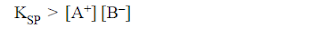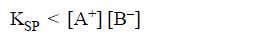Welcome to Chem Zipper.com......: SOLUBILITY(S) AND SOLUBILITY PRODUCT (Ksp)

## Wednesday, October 10, 2018

### SOLUBILITY(S) AND SOLUBILITY PRODUCT (Ksp)

Topics covered
(1)SOLUBILITY AND SOLUBILITY PRODUCT
(2) FACTER'S AFFECTING SOLUBILITY
(1) Effect of temperature on Solubility
(2) Effect of common ions on Solubility
(3) Effect of simultaneous on Solubility
(4) Effect of solvent on Solubility
(5) Effect of PH on Solubility
(i) Effect of PH on Solubility of metal hydroxide
(ii) Effect of PH on Solubility of salt of weak acid
(iii) Effect of PH on Solubility of salt of strong acid
(6) Effect of buffer solution on Solubility of salt
(7) Effect of complex formation on Solubility

SOLUBILITY(S):
Solubility of any substance (electrolyte) in solvent represent its amount present in the given amount of solution in order to make the solution saturated at a constant temperature.
MOLAR SOLUBILITY:
Number of mole of a solute dissolved in one litre saturated solution at constant temperature is called molar solubility.
Unit of solubility(s)=mole/litre
If solubility is equal or more than 0.1 then salt is soluble.
If solubility is equal or less than 0.1 then salt is partially soluble or sparingly soluble.
The solubility of substance in water may be classified as.
(1)Molecular Solubility: define for molecular solid, example glucose ,urea  and other covalent soluble solid.
(2)Ionic Solubility: define for electrolyte

SOLUBILITY PRODUCT (Ksp):
It is the product of the ionic concentration of the ions of binary solid electrolyte in saturated state at constant temperature.
(1) Let solubility of a compound Ax By be s moles L–1 it means that if more than s moles are dissolved in solvent (one litre )  only s moles will be soluble, rest will be insoluble, following equilibrium is established.
(2) According to law of mass action -
KSP is called solubility product.
(3) At a certain temperature solubility product of a compound is constant, it means that ions are formed in the manner that product of their concentration is always a constant. However, it becomes clear that if one of ions (A+ or B) is added from outside, it would tend to increase KSP because [A+] or [B] has increased, so that extra ions will react with other ions to convert into insoluble part and it precipitates.
(4)
Case-1 I
It means it is a saturated solution.
Case-2 IfIt means more solute can dissolved.
Case-3It means solution is supersaturated i.e. precipitation will start to occurs.

RELATIONSHIP BETWEEN SOLUBILITY AND SOLUBILITY PRODUCT:

The equilibrium for a saturated solution of a salt AxBy may be expressed as.
Let the solubility of the salt AxBy in water at a particular temperature be ‘s’ moles per litre then

NOTE-  (i) Ksp is the equilibrium constant which depends upon temperature only.
(ii)In general while calculating molar solubility we neglect hydrolysis of ions.

EXAMPLE(1): The solubility of CaCOis 0.03 g/L calculate Ksp ?
SOLUTION: solubility in term of mole /liter is 0.03/100 mole/liter (M wt of CaCO3 is 100 u)
we know that
Ksp =(s)2
= (0.03/100)2
=9 x 10-8

(1)AB type of salts:
(2)AB2 or A2B type of salts:

(3)AB3 or A3B type of salt:

(4)A2B3 type of salts:

EXAMPLE(2):  The solubility product expression for La2(CO3)3 is Ksp =?
SOLUTION:First, write the equilibrium expression. The subscript following each atom in the solid is the number of ions of that atom in solution. The total charges must add to zero:
Given the information above and the equilibrium expression:
EXAMPLE(2): What will be the solubility in moles/litre if solubility product of F3B2 is 2 × 10–30.
SOLUTION:

(2) FACTER'S AFFECTING SOLUBILITY
(1) Effect of temperature on Solubility: In General,most case Solubility increases on increasing temperature.However we must follow two case
(1) in endothermic reactions